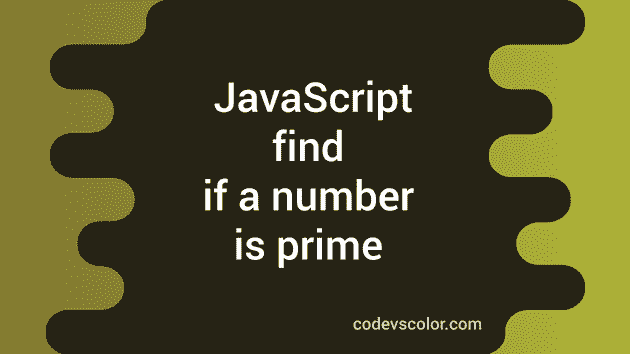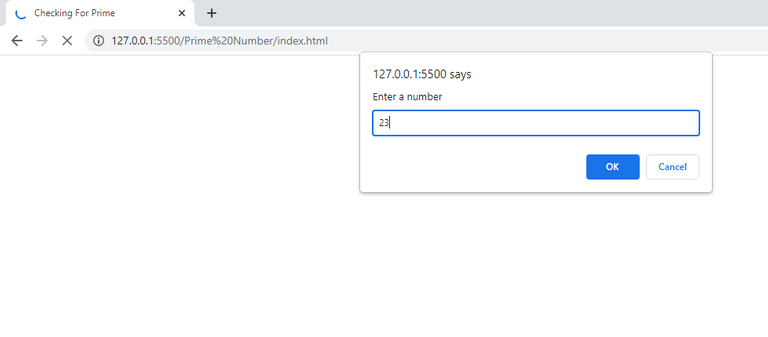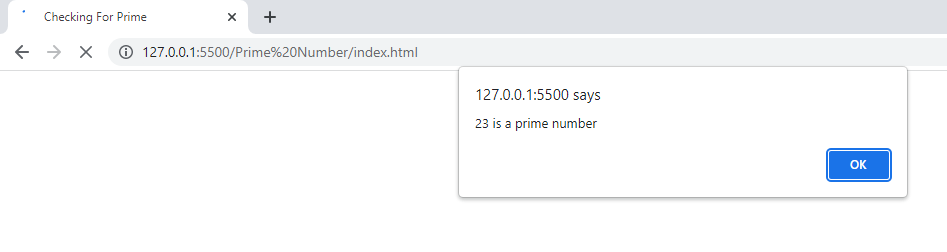# JavaScript Program To Check For Prime Or Composite Number

in STEMGeeks2 years agoImage Source

Of all the coding challenges in every programming language, finding if a number is prime or not is still my favorite one. There's a story behind it as well. While I joined my Bachelor's degree in Computer Science, I had to study C programming in my first semester. And this question came during my college first terminal exam. I could literally do every coding questions asked including pattern-printing, simple mathematical tasks including basic if-else condition but stumbled across this same coding question and I couldn't solve it like for half an hour. So the time came when I saw my friend leaving the exam hall submitting the paper and at the same time I asked the exam invigilator if I could go to the toilet🤣🤣.

After I was granted permission, I told my friend to come to toilet to help me with the coding. And he gave me this simple logic, you declare a variable count and initialize it to 0. Prime numbers have factor 1 and the number itself. So you use a for loop to iterate over the entered number and inside for-loop use if condition to increment the value of count whenever the loop finds the remainder to be 0. So what it means is that if a number is prime the value of count will always be 2. You then come out of that if block and again use another if-else to check if count value is 2. I thought for a moment like why such simple algorithm didn't came to my mind after that🤣. The same logic/algorithm has been implemented in JavaScript below.

``````<!DOCTYPE html>
<html>
<title>Checking For Prime</title>
<script>
var user_input = prompt("Enter a number");
var count = 0;
if (user_input ==1)
{
window.alert("1 is neither a prime number nor composite number.");
}
else{
for (var i=1; i<=user_input; i++)
{
if (user_input%i==0)
{
count = count + 1;
}
}

if (count == 2)
{
window.alert(user_input + " is a prime number")
}
else
{
window.alert(user_input + " is a composite number")
}
}

</script>
<body>
</body>
</html>
``````

While running the code, you will get a simple dialog popup box asking you to enter a value since we have used `prompt()` function in the above code.We wanted to check for a number which is 23. And it will show it is a prime number with another dialog popup box as we have used `alert()` function in above code to display the message.